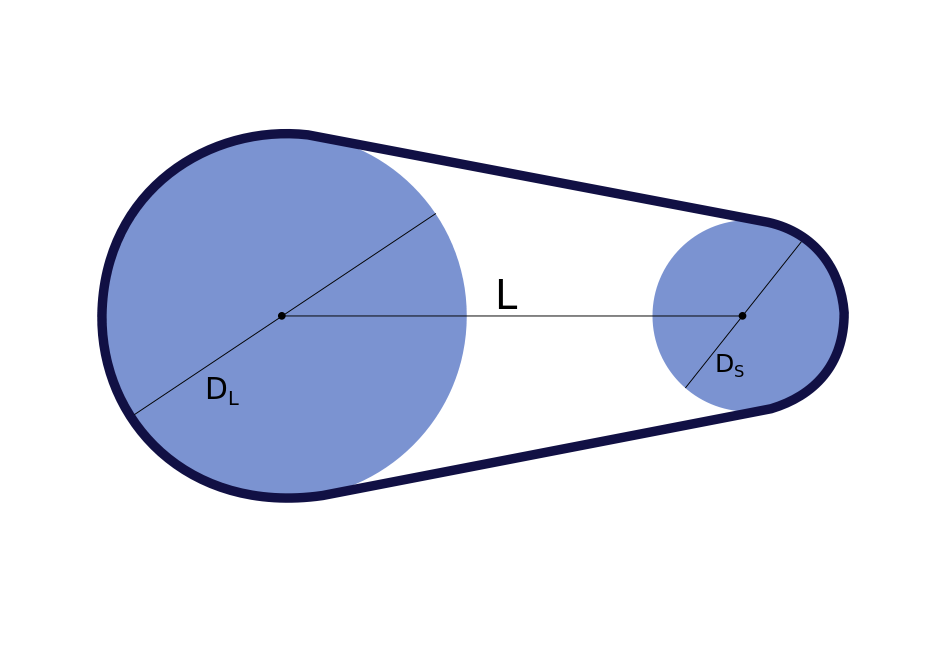# Belt Length Calculator

Created by Kenneth Alambra
Last updated: Jul 24, 2022

If you need to know the belt size for any two-pulley system, this belt length calculator is for you. Keep on reading to start learning:

• The importance of having the correct belt length;
• How to calculate belt length using the belt length formula; and
• How to use this belt length calculator.

## Importance of having the correct belt length

Belts that connect two pulleys together must have their specified tension to maintain the necessary friction between the belt and the pulleys to transmit torque successfully. We can use belts to link two pulleys of the same or different sizes, and we always do so on pulleys of the same surface, may they be flat, ribbed, toothed, or v-grooved.

Using longer belts than needed could result in the belts getting detached from the assembly. On the other hand, excessively forcing a short belt to fit a pulley system can lead to premature breakage of the belt. That is why we need to know precisely the correct belt length, just like getting the right gear sizes for a gear train.

In the next section of this text, we'll discuss how to calculate belt lengths.

## How to calculate belt lengths

To calculate the belt length (we denote in this text as $L_\text{belt}$) of a belt for a two pulley system, we only need to know three measurements, as shown in the illustration below:where:

• $D_L$ is the diameter of the large pulley;
• $L$ is the distance between the pulley axles; and
• $D_S$ is the diameter of the smaller pulley.

We will also need this belt length formula:

\scriptsize\ \begin{align*} L_\text{belt} &= \frac{\pi\times(D_L+D_S)}{2}\\ &+\ (D_L-D_S)\times\arcsin{\left(\frac{D_L-D_S}{2\times L}\right)}\\\\ &+\ 2\times\sqrt{L^2 - \frac{(D_L-D_S)^2}{4}} \end{align*}

You can also use this formula (common to engineers) to quickly approximate belt lengths for two-pulley systems with pulleys that have slight differences in sizes:

\scriptsize\ \begin{align*} L_\text{belt} &= \frac{\pi\times(D_L+D_S)}{2}\\ &+\ 2\times L + \frac{(D_L-D_S)^2}{4\times L} \end{align*}

🙋 Note that using the approximation formula above for pulleys with a huge difference in size could result in shorter belt lengths than needed.

## How to use this calculator

Keeping in mind a very long belt length formula to determine a belt length can be cumbersome and could lead to miscalculations, especially if you have to compute a few belt lengths. That is why we have this belt length calculator.

Let's say we need a belt for a v-groove pulley system with pulley diameters equal to 12 and 8 inches with their axles at a distance of 13 inches. Here is how to find the v-belt length using our tool:

1. Enter the diameter of the large pulley, which is 12 inches.
2. Key in the diameter of the small pulley. In this case, 8 inches.
3. Finally, input the pulley center distance of 13 inches. Upon doing so, our tool will display a v-belt length of 57.72 inches.

You can also click on the Advanced mode button below our calculator widget to display the engineering approximation of the belt length. For this example, you should see the same value of 57.72 inches.

Kenneth AlambraDiameter of the large pulley
in
Diameter of the small pulley
in
Pulley centre distance
in
Belt length
in
People also viewed…

### Engine displacement

Use this tool to calculate an engine displacement in cc, cubic inch, or any other unit.